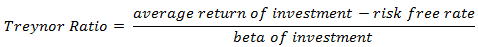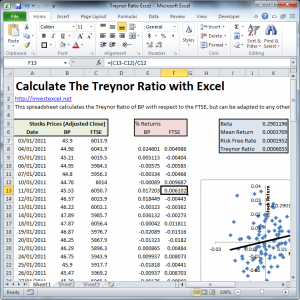Treynor Ratio – Guide and Spreadsheet

The Treynor Ratio is a performance metric that measures the effective return adjusted for market risk and is defined by the following equation.The investment beta measures the investment volatility relative to the market volatility. This is known as the systematic risk, or the risk associated with the entire market.

Systematic risk cannot be reduced by diversification and arises from changes in the business cycle, the political climate or economic policy.The Treynor Ratio (also known as the reward-to-volatility ratio) has a definition similar to that of the Sharpe Ratio – both are the effective return divided by the risk.  However, the Sharpe Ratio divides by the standard deviation (i.e. the total risk).  But the Treynor Ratio divides by the beta (the risk inherent in the market).  Well-diversified portfolios should have similar Sharpe and Treynor Ratios because the standard deviation reduces to the beta (i.e. the total risk and the systematic risk are the same).

The Treynor Ratio is negative if

• the risk free rate is greater than the expected return, and the beta is positive. This means that the fund manager has performed badly, taking on risk but failing to get performance better than the risk free rate
• or the risk-free rate is less than the expected return, but the beta is negative. This means that the fund manger has performed well, managing to reduce risk but getting a return better than the risk free rate

The primary advantage to the Treynor Ratio is that it indicates the volatility a stock brings to an entire portfolio.  The Treynor Ratio should be used only as a ranking mechanism for investments within the same sector.  When presented with investments that have the same return, investments with higher Treynor Ratios are less risky and better managed.1 thought on “Treynor Ratio – Guide and Spreadsheet”

1. How do you get the cell K7?

It’s the one with the formula =1.05^(1/250)-1.
Is it the average of our Benchmark monthly returns?
Or where does the 1.05 value come from?

I assume the (1/250) is to calculate the compound return.
The -1 is to change it to percentage format.
So the only problem is to know where does the 1.05 comes from.

Thanks for your time. Hope to hear from you soon.